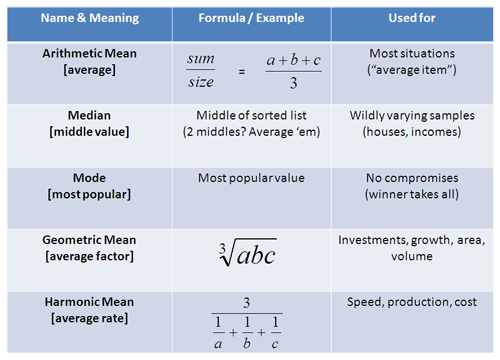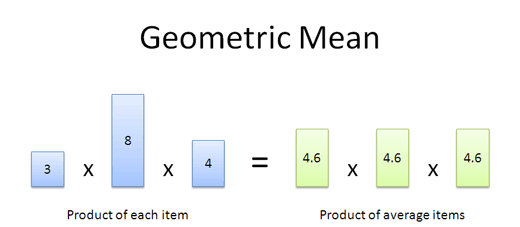# How to find the mean of a set of numbersThe average is a simple term with several meanings. The type of average to use depends on whether you’re adding, multiplying, grouping or dividing work among the items in your set.

Quick quiz: You drove to work at 30 mph, and drove back at 60 mph. What was your average speed?

Hint: It’s not 45 mph, and it doesn’t matter how far your commute is. Read on to understand the many uses of this statistical tool.## Geometric Mean

The “average item” depends on how we use our existing elements. Most of the time, items are added together and the arithmetic mean works fine. But sometimes we need to do more. When dealing with investments, area and volume, we don’t add factors, we multiply them.

Let’s try an example. Which portfolio do you prefer, i.e. which has a better typical year?

• Portfolio A: +10%, -10%, +10%, -10%
• Portfolio B: +30%, -30%, +30%, -30%

They look pretty similar. Our everyday average (arithmetic mean) tells us they’re both rollercoasters, but should average out to zero profit or loss. And maybe B is better because it seems to gain more in the good years. Right?

Wrongo! Talk like that will get you burned on the stock market: investment returns are multiplied, not added! We can’t be all willy-nilly and use the arithmetic mean — we need to find the actual rate of return:

• Portfolio A:
• Return: 1.1 * .9 * 1.1 * .9 = .98 (2% loss)
• Year-over-year average: (.98)^(1/4) = 0.5% loss per year (this happens to be about 2%/4 because the numbers are small).
• Portfolio B:
• 1.3 * .7 * 1.3 * .7 = .83 (17% loss)
• Year-over-year average: (.83)^(1/4) = 4.6% loss per year.
Related text  How to get better at basketball

A 2% vs 17% loss? That’s a huge difference! I’d stay away from both portfolios, but would choose A if forced. We can’t just add and divide the returns — that’s not how exponential growth works.Some more examples:

• Inflation rates: You have inflation of 1%, 2%, and 10%. What was the average inflation during that time? (1.01 * 1.02 * 1.10)^(1/3) = 4.3%
• Coupons: You have coupons for 50%, 25% and 35% off. Assuming you can use them all, what’s the average discount? (i.e. What coupon could be used 3 times?). (.5 * .75 * .65)^(1/3) = 37.5%. Think of coupons as a “negative” return — for the store, anyway.
• Area: You have a plot of land 40 × 60 yards. What’s the “average” side — i.e., how large would the corresponding square be? (40 * 60)^(0.5) = 49 yards.
• Volume: You’ve got a shipping box 12 × 24 × 48 inches. What’s the “average” size, i.e. how large would the corresponding cube be? (12 * 24 * 48)^(1/3) = 24 inches.

I’m sure you can find many more examples: the geometric mean finds the “typical element” when items are multiplied together. You take a set of numbers, multiply them, and take the Nth root (where N is the number of items you're considering).

I had wondered for a long time why the geometric mean was useful — now we know.

## Yikes, that was tricky

The harmonic mean is tricky: if you have separate machines running at 10 parts/hour and 20 parts/hour, then your average really is 15 parts/hour since each machine is independent and you are adding the capabilities. In that case, the arithmetic mean works just fine.

Related text  How to solve a rubiks cube

Sometimes it’s good to double-check to make sure the math works out. In the machine example, we claim to produce 7.14 widgets/hour. Ok, how long would it take to make 7.14 widgets?

• Prepping: 7.14 / 25 = .29 hours
• Finishing: 7.14 / 10 = .71 hours

And yes, .29 + .71 = 1, so the numbers work out: it does take 1 hour to make 7.14 widgets. When in doubt, try running a few examples to make sure your average rate really is what you calculated.

## Conclusion

Even a simple idea like the average has many uses — there are more uses we haven’t covered (center of gravity, weighted averages, expected value). The key point is this:

• The “average item” can be seen as the item that could replace all the others
• The type of average depends on how existing items are used (Added? Multiplied? Used as rates? Used as exclusive choices?)

It surprised me how useful and varied the different types of averages were for analyzing data. Happy math.